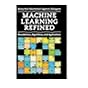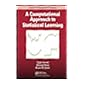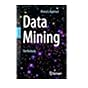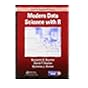Normal view MARC view ISBD view

# A computational approach to statistical learning

Material type:BookPublisher: Boca Raton CRC Press 2019Description: xiii, 361 p. Includes bibliographical references and index.ISBN: 9781138046375.DDC classification: 006.31015195 Summary: A Computational Approach to Statistical Learning gives a novel introduction to predictive modeling by focusing on the algorithmic and numeric motivations behind popular statistical methods. The text contains annotated code to over 80 original reference functions. These functions provide minimal working implementations of common statistical learning algorithms. Every chapter concludes with a fully worked out application that illustrates predictive modeling tasks using a real-world dataset. The text begins with a detailed analysis of linear models and ordinary least squares. Subsequent chapters explore extensions such as ridge regression, generalized linear models, and additive models. The second half focuses on the use of general-purpose algorithms for convex optimization and their application to tasks in statistical learning. Models covered include the elastic net, dense neural networks, convolutional neural networks (CNNs), and spectral clustering. A unifying theme throughout the text is the use of optimization theory in the description of predictive models, with a particular focus on the singular value decomposition (SVD). Through this theme, the computational approach motivates and clarifies the relationships between various predictive models. https://www.crcpress.com/A-Computational-Approach-to-Statistical-Learning/Arnold-Kane-Lewis/p/book/9781138046375
Tags from this library: No tags from this library for this title.
average rating: 0.0 (0 votes)
Item type Current location Item location Collection Call number Status Date due Barcode
Books Vikram Sarabhai Library
General Stacks
Slot 104 (0 Floor, West Wing) Non-fiction 006.31015195 A7C6 (Browse shelf) Not for Issue 199731
##### Browsing Vikram Sarabhai Library Shelves , Shelving location: General Stacks , Collection code: Non-fiction Close shelf browser006.31 S8R3 Reinforcement learning: an introduction 006.31 V2 Variants of evolutionary algorithms for real-world applications 006.31 W2M2 Machine learning refined: foundations, algorithms, and applications 006.31015195 A7C6 A computational approach to statistical learning 006.31068403 K2M2 Machine learning for decision makers: cognitive computing fundamentals for better decision making 006.312 A4D2 Data mining: the textbook 006.312 B2M6 Modern data science with R

Table of contents:

1. Introduction

Computational approach

Statistical learning

Example

Prerequisites

How to read this book

Supplementary materials

Formalisms and terminology

Exercises

2. Linear Models

Introduction

Ordinary least squares

The normal equations

Solving least squares with the singular value decomposition

Directly solving the linear system

(*) Solving linear models with orthogonal projection

(*) Sensitivity analysis

(*) Relationship between numerical and statistical error

Implementation and notes

Application: Cancer incidence rates

Exercises

3. Ridge Regression and Principal Component Analysis

Variance in OLS

Ridge regression

(*) A Bayesian perspective

Principal component analysis

Implementation and notes

Application: NYC taxicab data

Exercises

4. Linear Smoothers

Non-linearity

Basis expansion

Kernel regression

Local regression

Regression splines

(*) Smoothing splines

(*) B-splines

Implementation and notes

Application: US census tract data

Exercises

5. Generalized Linear Models

Classification with linear models

Exponential families

Iteratively reweighted GLMs

(*) Numerical issues

(*) Multi-class regression

Implementation and notes

Application: Chicago crime prediction

Exercises

6. Additive Models

Multivariate linear smoothers

Curse of dimensionality

Additive models

(*) Additive models as linear models

(*) Standard errors in additive models

Implementation and notes

Application: NYC flights data

Exercises

7. Penalized Regression Models

Variable selection

Penalized regression with the `- and `-norms

Orthogonal data matrix

Convex optimization and the elastic net

Coordinate descent

(*) Active set screening using the KKT conditions

(*) The generalized elastic net model

Implementation and notes

Application: Amazon product reviews

Exercises

8. Neural Networks

Dense neural network architecture

Stochastic gradient descent

Backward propagation of errors

Implementing backpropagation

Recognizing hand written digits

(*) Improving SGD and regularization

(*) Classification with neural networks

(*) Convolutional neural networks

Implementation and notes

Application: Image classification with EMNIST

Exercises

9. Dimensionality Reduction

Unsupervised learning

Kernel functions

Kernel principal component analysis

Spectral clustering

t-Distributed stochastic neighbor embedding (t-SNE)

Autoencoders

Implementation and notes

Application: Classifying and visualizing fashion MNIST

Exercises

10. Computation in Practice

Reference implementations

Sparse matrices

Sparse generalized linear models

Computation on row chunks

Feature hashing

Data quality issues

Implementation and notes

Application

Exercises

A Matrix Algebra

A Vector spaces

A Matrices

A Other useful matrix decompositions

B Floating Point Arithmetic and Numerical Computation

B Floating point arithmetic

B Numerical sources of error

B Computational effort

A Computational Approach to Statistical Learning gives a novel introduction to predictive modeling by focusing on the algorithmic and numeric motivations behind popular statistical methods. The text contains annotated code to over 80 original reference functions. These functions provide minimal working implementations of common statistical learning algorithms. Every chapter concludes with a fully worked out application that illustrates predictive modeling tasks using a real-world dataset.

The text begins with a detailed analysis of linear models and ordinary least squares. Subsequent chapters explore extensions such as ridge regression, generalized linear models, and additive models. The second half focuses on the use of general-purpose algorithms for convex optimization and their application to tasks in statistical learning. Models covered include the elastic net, dense neural networks, convolutional neural networks (CNNs), and spectral clustering. A unifying theme throughout the text is the use of optimization theory in the description of predictive models, with a particular focus on the singular value decomposition (SVD). Through this theme, the computational approach motivates and clarifies the relationships between various predictive models.

https://www.crcpress.com/A-Computational-Approach-to-Statistical-Learning/Arnold-Kane-Lewis/p/book/9781138046375

There are no comments for this item.

Log in to your account to post a comment.

Powered by Koha